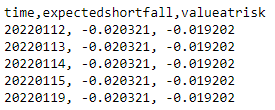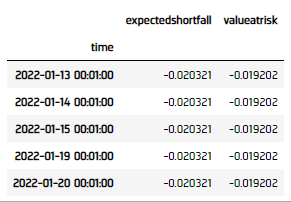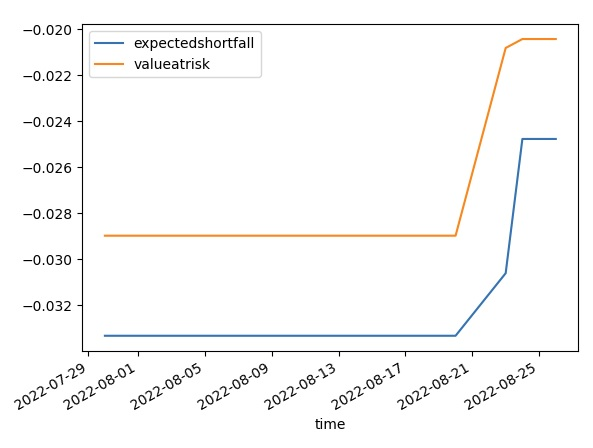# Indicators

## Custom Indicators

### Create Subscriptions

You need to subscribe to some market data in order to calculate indicator values.

var qb = new QuantBook();
var symbol = qb.AddEquity("SPY").Symbol;
qb = QuantBook()
symbol = qb.AddEquity("SPY").Symbol

### Create Indicator Timeseries

You need to subscribe to some market data in order to calculate a timeseries of indicator values.

Follow these steps to create an indicator timeseries:

1. Get some historical data.
2. var history = qb.History(symbol, 70, Resolution.Daily);
history = qb.History[TradeBar](symbol, 70, Resolution.Daily)
3. Define a custom indicator class that inherits from the Indicator superclass.
4. Define a custom indicator class. Note the PythonIndicator superclass inheritance, Value attribute, and Update method are mandatory.
5. In this tutorial, create an ExpectedShortfallPercent indicator that uses Monte Carlo to calculate the expected shortfall of returns. Use the WindowIndicator superclass instead of Indicator for using a period of historical data stored in a RollingWindow.

In this tutorial, create an ExpectedShortfallPercent indicator that uses Monte Carlo to calculate the expected shortfall of returns.

public class ExpectedShortfallPercent : WindowIndicator<IndicatorDataPoint>, IIndicatorWarmUpPeriodProvider
{
private decimal _alpha;

// Set up IndicatorDataPoint attributes for the indicator.
public IndicatorBase<IndicatorDataPoint> ValueAtRisk { get; }

// Set up the WarmUpPeriod attribute to provide implementation of the IIndicatorWarmUpPeriodProvider interface.
public override int WarmUpPeriod => Period;

// Set up the constructor.
// period: The lookback period for return distribution.
// alpha: Alpha level of VaR cutoff.
public ExpectedShortfallPercent(int period, decimal alpha)
: base("ES", period)
{
_alpha = alpha;
ValueAtRisk = new Identity("ES_VaR");
}

// Override the IsReady method to set up the flag of the Indicator and its IndicatorDataPoint attributes are ready.

// Mandatory: Override the ComputeNextValue method to calculate the indictor value.
protected override decimal ComputeNextValue(IReadOnlyWindow<IndicatorDataPoint> window, IndicatorDataPoint input)
{
if (Samples < 2)
return 0m;

var n = Math.Min(Period, Samples);
var cutoff = (int) Math.Ceiling(n * _alpha);

var samples = new List<decimal>();
for (int i = 0; i < window.Count - 1; i++)
{
samples.Add( (window[i] - window[i+1]) / window[i+1] );
}
var lowest = samples.OrderBy(x => x).Take(cutoff);

ValueAtRisk.Update(input.Time, lowest.Last());
return lowest.Average();
}
}
class ExpectedShortfallPercent(PythonIndicator):
import math, numpy as np

def __init__(self, period, alpha):
self.Value = None   # Attribute represents the indicator value
self.ValueAtRisk = None

self.alpha = alpha

self.window = RollingWindow[float](period)

# Override the IsReady attribute to flag all attributes values are ready.
@property
return self.Value and self.ValueAtRisk

# Method to update the indicator values. Note that it only receives 1 IBaseData object (Tick, TradeBar, QuoteBar) argument.
def Update(self, input: BaseData) -> bool:
count = self.window.Count

# Update the Value and other attributes as the indicator current value.
if count >= 2:
cutoff = math.ceil(self.alpha * count)

ret = [ (self.window[i] - self.window[i+1]) / self.window[i+1] for i in range(count-1) ]
lowest = sorted(ret)[:cutoff]

self.Value = np.mean(lowest)
self.ValueAtRisk = lowest[-1]

# return a boolean to indicate IsReady.
return count >= 2
6. Initialize a new instance of the custom indicator.
7. var es = new ExpectedShortfallPercent(50, 0.05m);
custom = ExpectedShortfallPercent(50, 0.05)
8. Create a RollingWindow for each attribute of the indicator to hold their values.
9. In this example, save 20 data points.

var time = new RollingWindow<DateTime>(20);
var window = new Dictionary<string, RollingWindow<decimal>>();
window["expectedshortfall"] = new RollingWindow<decimal>(20);
window["valueatrisk"] = new RollingWindow<decimal>(20);

window = {}
window['time'] = RollingWindow[DateTime](20)
window['expectedshortfall'] = RollingWindow[float](20)
window['valueatrisk'] = RollingWindow[float](20)

10. Attach a handler method to the indicator that updates the RollingWindow objects.
11. es.Updated += (sender, updated) =>
{
var indicator = (ExpectedShortfallPercent) sender;
};

When the indicator receives new data, the preceding handler method adds the new IndicatorDataPoint values into the respective RollingWindow.

12. Iterate through the historical market data and update the indicator.
13. foreach(var bar in history){
es.Update(bar.EndTime, bar.Close);
}
for bar in history:
custom.Update(bar)

# The Updated event handler is not available for custom indicator in Python, RollingWindows are needed to be updated in here.
window['valueatrisk'].Add(custom.ValueAtRisk)
14. Display the data.
15. Console.WriteLine($"time,{string.Join(',', window.Select(kvp => kvp.Key))}"); foreach (var i in Enumerable.Range(0, 5).Reverse()) { var data = string.Join(", ", window.Select(kvp => Math.Round(kvp.Value[i],6))); Console.WriteLine($"{time[i]:yyyyMMdd}, {data}");
}16. Populate a DataFrame with the data in the RollingWindow objects.
17. custom_dataframe = pd.DataFrame(window).set_index('time'))### Plot Indicators

Jupyter Notebooks don't currently support libraries to plot historical data, but we are working on adding the functionality. Until the functionality is added, use Python to plot custom indicators.

Follow these steps to plot the indicator values:

1. Call the plot method.
2. custom_dataframe.plot()
3. Show the plot.
4. plt.show()You can also see our Videos. You can also get in touch with us via Discord.